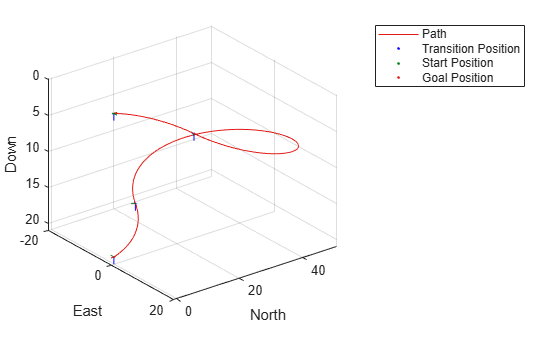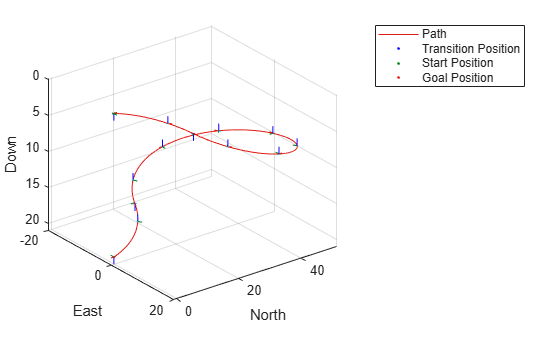# interpolate

Interpolate poses along UAV Dubins path segment

## Syntax

``poses = interpolate(pathSegObj,lengths)``

## Description

example

````poses = interpolate(pathSegObj,lengths)` interpolates poses along the path segment at the specified path lengths. Transitions between motion types are always included.```

## Examples

collapse all

This example shows how to connect poses using the `uavDubinsConnection` object and interpolate the poses along the path segment at the specified path lengths.

Connect Poses Using UAV Dubins Connection Path

Create a `uavDubinsConnection` object.

`connectionObj = uavDubinsConnection;`

Define start and goal poses as [`x`, `y`, `z`, `headingAngle`] vectors.

```startPose = [0 0 0 0]; % [meters, meters, meters, radians] goalPose = [0 0 20 pi];```

Calculate a valid path segment and connect the poses.

`[pathSegObj,pathCost] = connect(connectionObj,startPose,goalPose);`

Show the generated path.

`show(pathSegObj{1})`Interpolate the Poses

Specify the interval to interpolate along the path.

```stepSize = pathSegObj{1}.Length/10; lengths = 0:stepSize:pathSegObj{1}.Length;```

Interpolate the poses along the path segment at the specified path lengths.

`poses = interpolate(pathSegObj{1},lengths); % [x, y, z, headingAngle, flightPathAngle, rollAngle]`

Visualize the Transition Poses

Compute the translation and rotation matrix of the transition poses, excluding the start and goal poses. The `posesTranslation` matrix consists of the first three columns of the `poses` matrix specifying the position `x`, `y`, and `z`.

`posesTranslation = poses(2:end-1,1:3); % [x, y, z]`

Increment the elements of the fourth column of the `poses` matrix representing the `headingAngle` by `pi` and assign it as the first column of the rotation matrix `posesEulRot` in ZYX Euler angle representation. A column of `pi` and a column of zeros forms the second and the third columns of the `posesEulRot` matrix, respectively. Convert the `posesEulRot` matrix from Euler angles to quaternion and assign to `posesRotation`.

```N = size(poses,1)-2; posesEulRot = [poses(2:end-1,4)+pi,ones(N,1)*pi,zeros(N,1)]; % [headingAngle + pi, pi, 0] posesRotation = quaternion(eul2quat(posesEulRot,'ZYX'));```

Plot transform frame of the transition poses by specifying their translations and rotations using `plotTransforms`.

```hold on plotTransforms(posesTranslation,posesRotation,'MeshFilePath','fixedwing.stl','MeshColor','cyan')```## Input Arguments

collapse all

Path segment, specified as a `uavDubinsPathSegment` object.

Lengths along path to interpolate poses, specified as a positive numeric vector in meters.

For example, specify `0:stepSize:pathSegObj{1}.Length` to interpolate at the interval specified by stepSize along the path. Transitions between motion types are always included.

Data Types: `double`

## Output Arguments

collapse all

Interpolated poses along the path segment, returned as a six-element numeric matrix [x, y, z, headingAngle, flightPathAngle, rollAngle]. Each row of the matrix corresponds to a different interpolated pose along the path.

x, y, and z specify the position in meters. headingAngle, flightPathAngle, and rollAngle specify the orientation in radians.

## Version History

Introduced in R2019b